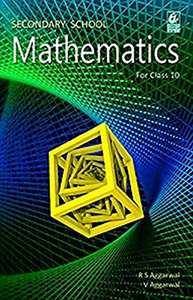Share

R.S. Aggarwal solutions for Class 10 Mathematics chapter 17 - Volumes and Surface Areas of Solids

Secondary School Mathematics for Class 10

Chapters

Chapter 1: Real Numbers

Chapter 2: Polynomials

Chapter 3: Linear Equations in Two Variables

Chapter 4: Quadratic Equations

Chapter 5: Arithmetic Progression

Chapter 6: Coordinate Geometry

Chapter 7: Triangles

Chapter 8: Circles

Chapter 9: Constructions

Chapter 10: Trignometric Ratios

Chapter 11: T-Ratios of Some Particular Angles

Chapter 12: Trigonometric Ratios of Some Complemantary Angles

Chapter 13: Trigonometric identities

Chapter 14: Heights and Distances

Chapter 15: Perimeter And Area of Plane Figures

Chapter 16: Area of Circle, Sector and Segment

Chapter 17: Volumes and Surface Areas of Solids

Chapter 18: Mean, Median, Mode of Grouped Data, Cumulative Frequency Graph and Ogive

Chapter 19: Probability

R.S. Aggarwal Secondary School Mathematics Class 10Chapter 17: Volumes and Surface Areas of Solids

Chapter 17: Volumes and Surface Areas of Solids solutions [Page 875]

Q 9 | Page 875

A military tent of height 8.25 m is in the form of a right circular cylinder of base diameter 30 m and height 5.5 m surmounted by a right circular cone of same base radius. Find the length of canvas used in making the tent, if the breadth of the canvas is 1.5 m.

Chapter 17: Volumes and Surface Areas of Solids solutions [Page 920]

Q 29 | Page 920

The slant height of a bucket is 45 cm and the radii of its top and bottom are 28 cm and 7 cm, respectively. The curved surface area of the bucket is

• 4953 cm

• 4952 cm

• 4951 cm

• 4950 cm

Chapter 17: Volumes and Surface Areas of Solids

R.S. Aggarwal Secondary School Mathematics Class 10R.S. Aggarwal solutions for Class 10 Mathematics chapter 17 - Volumes and Surface Areas of Solids

R.S. Aggarwal solutions for Class 10 Maths chapter 17 (Volumes and Surface Areas of Solids) include all questions with solution and detail explanation. This will clear students doubts about any question and improve application skills while preparing for board exams. The detailed, step-by-step solutions will help you understand the concepts better and clear your confusions, if any. Shaalaa.com has the CBSE Secondary School Mathematics for Class 10 solutions in a manner that help students grasp basic concepts better and faster.

Further, we at Shaalaa.com are providing such solutions so that students can prepare for written exams. R.S. Aggarwal textbook solutions can be a core help for self-study and acts as a perfect self-help guidance for students.

Concepts covered in Class 10 Mathematics chapter 17 Volumes and Surface Areas of Solids are Introduction of Surface Areas and Volumes, Surface Area of a Combination of Solids, Volume of a Combination of Solids, Conversion of Solid from One Shape to Another, Frustum of a Cone, Surface Areas and Volumes Examples and Solutions.

Using R.S. Aggarwal Class 10 solutions Volumes and Surface Areas of Solids exercise by students are an easy way to prepare for the exams, as they involve solutions arranged chapter-wise also page wise. The questions involved in R.S. Aggarwal Solutions are important questions that can be asked in the final exam. Maximum students of CBSE Class 10 prefer R.S. Aggarwal Textbook Solutions to score more in exam.

Get the free view of chapter 17 Volumes and Surface Areas of Solids Class 10 extra questions for Maths and can use Shaalaa.com to keep it handy for your exam preparation

S